+ Text Only Site
+ Non-Flash Version
+ Contact Glenn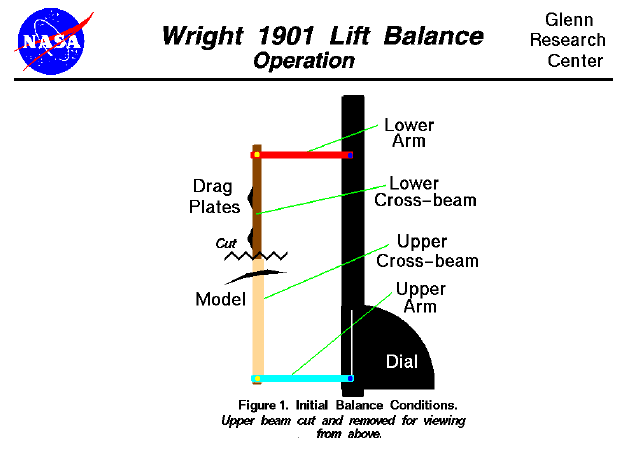During the fall of 1901, the brothers began to question the aerodynamic data on which they were basing their aircraft designs. They decided to conduct a series of wind tunnel tests to verify the results they were experiencing in flight. They would measure the aerodynamic lift and drag on small models of their wing designs using a wind tunnel in their bicycle shop in Dayton, Ohio. They built two separate balances to perform these measurements, one for lift. and the other for drag. On this page we will discuss the operation of the lift balance. Because of the form of the lift equation used by the brothers, they were interested in measuring the lift generated by a model relative to the drag of a reference flat plate. The operation of the balance depends on the concepts of torques and equilibrium. As the test begins, the tunnel is turned off and the upper and lower cross beams are moved so that the upper beam lies on top of the lower beam and the arms are parallel with the sides of the tunnel as shown in the figure at the top of this page. In this drawing, we are using the same color scheme as used on the page describing the design of the lift balance. We are looking down onto the balance from above and we have cut the upper beam (saw-toothed line) so that we can see the orientation of the lower beam and arm. The lower beam holds the reference drag plates, while the upper beam holds the model being tested. The fan which pushes air through the tunnel is far to the right of this drawing. The model is set to some desired angle of attack and the fan is turned on. The cross beams and arms move because of torques generated by the drag force of the plates and the lift and drag force of the model on the long axles. Because the friction sleeve is engaged, the upper and lower beams move together. When the torque created by the drag plates and the drag of the model is balanced by the torque generated by the lift of the model, the balance no longer moves and assumes the following orientation: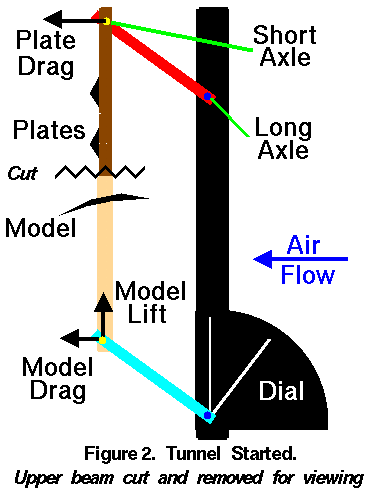We have again cut the upper beam so that you can see the lower beam and arm. On this figure we show the direction of the forces by the small arrows. We have placed the forces at the short axles because that is where the forces generated by the model and plates are transmitted to the arms. (Since the cross-beams are supported at both ends, and there are two arms at each end, each arm actually transmits one fourth of the force.) The arms transmit the force to the long axle and eventually to the frame and tunnel walls. As the arms rotate because of the torque, the pointer of the dial rotates as well. The pointer is always perpendicular to the lower arm. To eliminate the torque produced by the drag of the model, the friction sleeve is dis-engaged and the upper cross beam is moved until the arm is parallel to the side of the tunnel.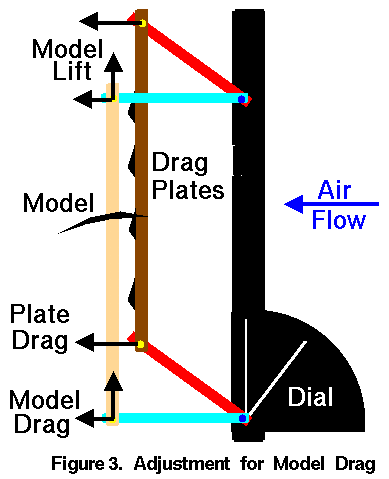The bottom cross beam does not move because the the friction sleeve is dis-engaged during the adjustment and the dial pointer continues to record the amount of rotation of the long axle. When the sleeve is re-engaged, the balance achieves a new equilibrium because of the torques of the plates and the torque of the model on the long axles. Several adjustments must be made until the upper arms remain parallel to the side of the tunnel. The torque about a point on an object is equal to the force applied to the object times the perpendicular distance from the point to the place where the force is applied. This figure shows the magnitude and orientation of the torques on the long axles.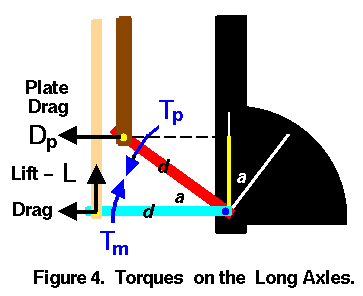Because the drag of the model is alligned with the long axle, the distance from the long axle measured perpendicular to the model drag force is zero, and the model drag does not exert any torque on the long axle. The distance from the long axle perpendicular to the lift force is the arm length (d). The direction of the lift force (L) generates a torque from the model (Tm) in the clockwise direction. Tm = L * d The distance from the long axle perpendicular to the plate drag force is shown on Figure 4. by a yellow line near the dial. The length of this line is the arm length (d) times the sine of the angle (a) at which the lower arm is inclined. The direction of the plate drag force (Dp) generates a torque from the plate (Tp) in the counter-clockwise direction. Tp = Dp * d * sin(a) In equilibrium, these torques are equal and the long axle does not rotate. Tm = Tp L * d = Dp * d * sin(a) sin(a) = L / Dp We can get a mathematical relation for the lift of the model from the lift equation. L= k * V^2 * Am * cl where (k) is the Smeaton coefficient, (V) is the velocity, (Am) is the surface area of the model, and (cl) is the lift coefficient. Similarly, for the reference plates the drag equation is: D= k * V^2 * Ap * cd where (Ap) is the area of the drag plates, and (cd) is the drag coefficient. Substituting into the torque equilibrium equation: sin(a) = (k * V^2 * Am * cl) / (k * V^2 * Ap * cd) For a flat plate, the drag coefficient is equal to 1.0. Cancelling out similar variables we get cl = sin(a) * Ap / Am Notice that the angle (a) between the upper and lower arms is also the angle recorded on the lift dial from the vertical reference line to the pointer attached to the long axle. Knowing the area of the drag plates and area of the model, the Wrights determined the lift coefficient by recording the angle on the dial and solving the equation given above. They repeated the test process many times, changing models and angle of attack of the models to generate graphs of their results. You can do the same thing, on-line, with an interactive computer simulation of the the tunnel and balance which we have developed. Observations There are several interesting aspects to the operation of the balance. At the time the brothers began testing, they doubted both the value of Smeaton's pressure coefficient (k) and the data curves produced by Otto Lilienthal that used the Smeaton coefficient to determine cl. The two problems seemed to be locked together. But notice that the equation for determining the lift coefficient from the measured angle contains no reference to Smeaton's coefficient. By balancing the model lift against the flat plate drag, the brothers were able to separate the two problems. They were then able to determine their own value for the Smeaton coefficient based on their kite and glider experiments, and their own (correct) values for the lift coefficient from the 1901 wind tunnel. It is also interesting to note the role that the area ratio plays in the lift coefficient equation. The maximum value for the sin(a) is 1.0 (at a = 90 degrees). Without the area ratio, one could never get a lift coefficient greater than 1.0. Or to put it another way, with the area of the plates equal to the area of the model, if the lift of the model is greater than the drag of the plates (cl > 1.0), the upper beam can not be adjusted to remove the drag of model; it won't balance with the upper arm parallel to the side of the tunnel. On the brothers' balance, the total area of the drag plates was 8 square inches. The area of the models ranged from 3 to 8 square inches, with most of them at 6 square inches. Several of the 6 square inch models had lift coefficients greater than 1.0, which initially surprised Wilbur Wright. Navigation..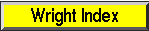Beginner's Guide Home Page+ Inspector General Hotline + Equal Employment Opportunity Data Posted Pursuant to the No Fear Act + Budgets, Strategic Plans and Accountability Reports + Freedom of Information Act + The President's Management Agenda + NASA Privacy Statement, Disclaimer, and Accessibility CertificationEditor: Nancy Hall NASA Official: Nancy Hall Last Updated: May 13 2021 + Contact Glenn